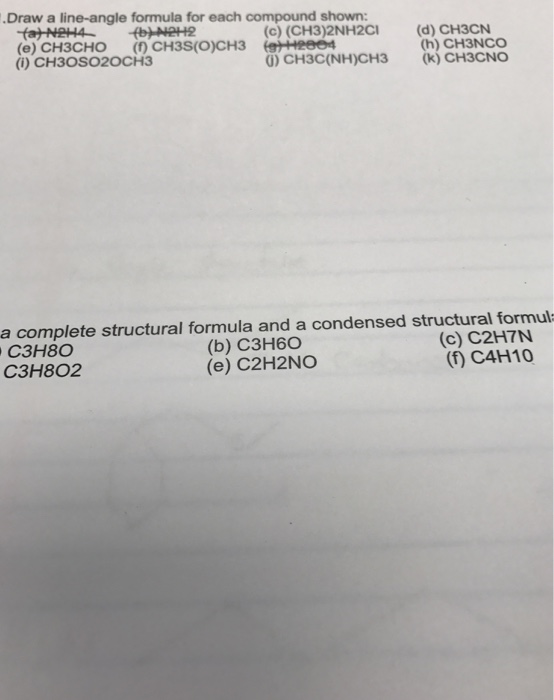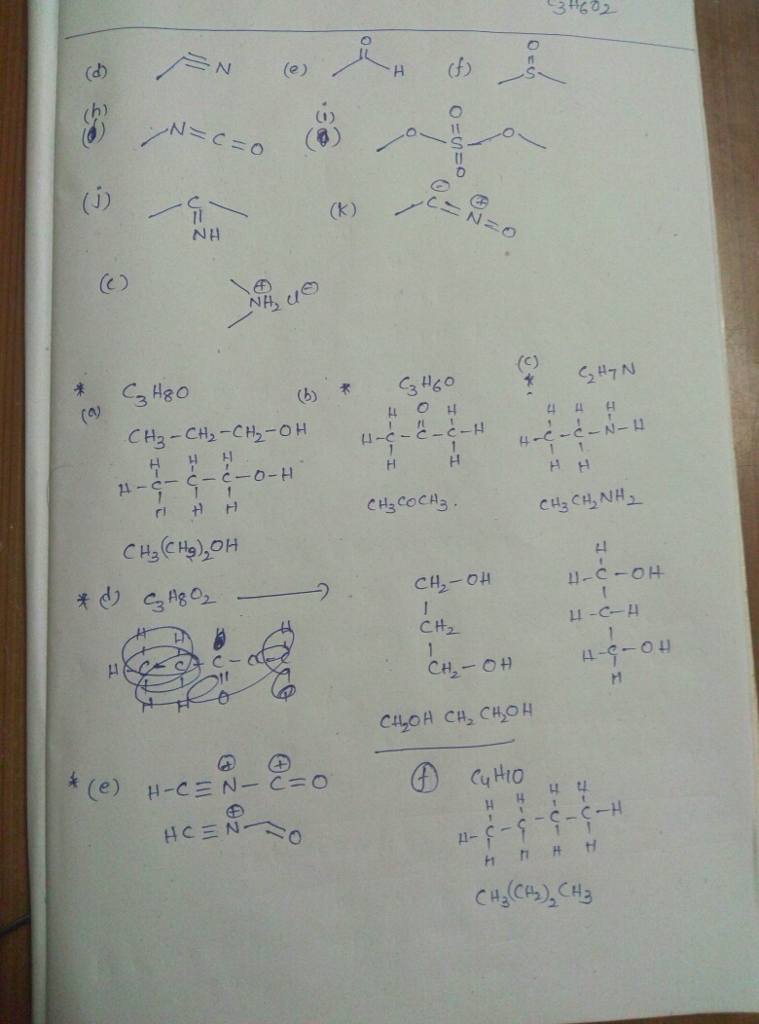# Question & Answer: Draw a line-angle formula for each compound shown: (a) N2H4 (b) N2H2 (c) (CH3)…..Draw a line-angle formula for each compound shown: (a) N2H4 (b) N2H2 (c) (CH3)_2NH2CL (d) CH3CN (e) CH3CHO (f)CH3S(O)CH3 (g)H2SO4 (h) CH3NCO (i) CH3OSO2OCH3 (j) CH3C(NH)CH3 (k) CH3CNO a complete structural formula and a condensed structural formul C3H8O (b) C3H6O (c) C2H7N C3H802 (e) C2H2NO (f) C4H10